### Avail Impeccable ME606 Z-Transforms and Filters Concepts Assignment Help and Assessment Help Services At Best Prices!!

Home   Course
Previous << || >> Next

DONT MISS YOUR CHANCE TO EXCEL IN ME606 Z-TRANSFORMS AND FILTERS CONCEPTS ASSIGNMENT! HIRE TUTOR OF EXPERTSMINDS.COM FOR PERFECTLY WRITTEN ME606 DIGITAL SIGNAL PROCESSING ASSIGNMENT SOLUTIONS!

DIGITAL SIGNAL PROCESSING

Assessment - z-Transforms, Filters Concepts

Learning Outcomes -

a. Develop and implement signal processing algorithms in Matlab

b. Undertake in-depth design of digital filters

INTRODUCTION

The Aim of this assignment is to develop and analyse the FIR filter designing and Z transformation.The filters like low pass,band pass and bandstop signals are analyzed.The impulse response and frequency  response of the filters are plotted.ROC curve of Z transform is generated.To design the filters frequency sampling approach and windowed fourier series approach is used.

1-1 Designing a low pass FIR filter using Windowed Fourier Series approach

Question 1) Run the above program and plot the impulse response and the amplitude spectrum of the filter.

MATLAB Code

wp=pi/8; % lowpass filter bandwidth

M=121;

n=-(M-1)/2:(M-1)/2; % selection time window

h0=(wp/pi)*sinc((wp/pi)*n); % truncated impulse response

h=h0.*rectwin(M)'; % windowing

% h=h0.*hamming(M)';% windowing

% h=h0.*hanning(M)';% windowing

% h=h0.*bartlett(M)';% windowing

% h=h0.*blackman(M)';% windowing

figure;plot(h)

ylabel('Impulse response')

xlabel('Samples')

% spectrum

FFTsize=512;

pxx=20*log10(abs(fft(h,FFTsize)));

fxx=(0:(FFTsize/2)-1)*(pi/(FFTsize/2));

figure;plot(fxx,pxx(1:FFTsize/2));

ylabel('Amplitude[dB]');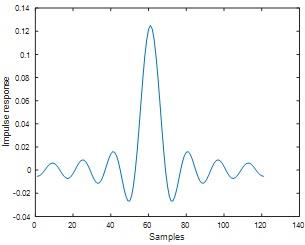Fig 1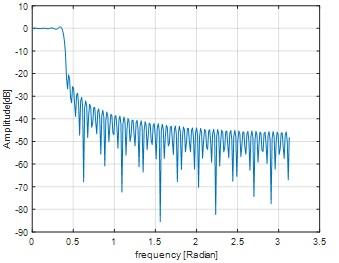Fig 2

Question 2) Measure the filter frequency response at ωp = pi/8. Is it close to the expected value of -6dB? How much is the maximum ripple in the pass band.

MATLAB Code

lp=designfilt('lowpassfir','Filterorder',80,'CutoffFrequency',pi/8);

freqz(lp);

[fp wp]=freqz(lp);

figure

plot(wp/pi,db(fp));

ylabel('Magnitude (dB)')

title('Frequency Response')

grid on

%% pass band rible

ps=0.02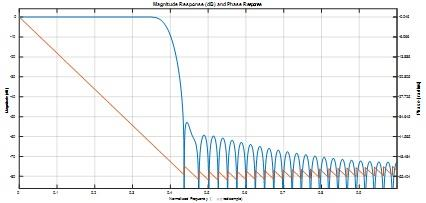Fig 3

No , it's not close to -6 dB

Maximum pass band ripple is 0.01 dB

Question 3) This filtering technique does not define any cutoff. If we assume the stopband starts at -20dB, measure the ratio of the transient band to the pass band.

Ratio of transition band to passband=(stop band frequency of the filter- pass band frequency of the filter)/(pass band frequency of the filter)

From fig 2 we can seen that

Stop band frequency at -20 dB is 0.4

Transient band frequency is 0.4-0.35=0.05

Pass band frequency is  0.35

Ratio of transition band to passband = (0.4- 0.35)/0.35

=0.1429

GET GUARANTEED SATISFACTION OR MONEY BACK UNDER ME606 Z-TRANSFORMS AND FILTERS CONCEPTS ASSIGNMENT HELP SERVICES OF EXPERTSMINDS.COM - ORDER TODAY NEW COPY OF THIS ASSIGNMENT!

Question 4) Increase the filter impulse response length to M = 255 and run the program.

MATLAB Code

wp=pi/8; % lowpass filter bandwidth

M=255;

n=-(M-1)/2:(M-1)/2; % selection time window

h0=(wp/pi)*sinc((wp/pi)*n); % truncated impulse response

h=h0.*rectwin(M)'; % windowing

% h=h0.*hamming(M)';% windowing

% h=h0.*hanning(M)';% windowing

% h=h0.*bartlett(M)';% windowing

% h=h0.*blackman(M)';% windowing

figure;plot(h)

ylabel('Impulse response')

xlabel('Samples')

% spectrum

FFTsize=512;

pxx=20*log10(abs(fft(h,FFTsize)));

fxx=(0:(FFTsize/2)-1)*(pi/(FFTsize/2));

figure;plot(fxx,pxx(1:FFTsize/2));

ylabel('Amplitude[dB]');

lp=designfilt('lowpassfir','Filterorder',80,'CutoffFrequency',pi/8);

freqz(lp);

[fp wp]=freqz(lp);

figure

plot(wp/pi,db(fp));

ylabel('Magnitude (dB)')

title('Frequency Response')

grid on

ps=0.01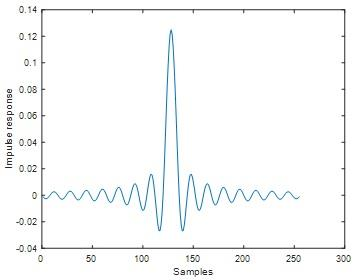Fig 4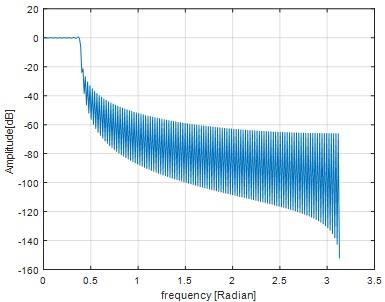Fig 5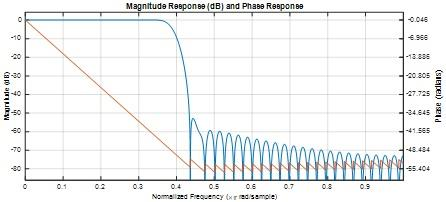Fig 6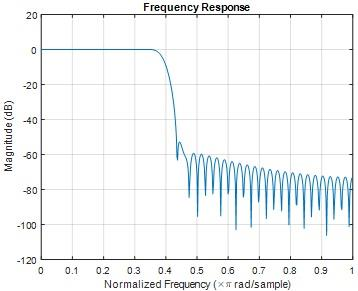Fig 7

If we assume the stopband starts at -20dB, measure the ratio of the transient band to the passband.

a)

Ratio of transition band to passband=(stop band frequency of the filter- pass band frequency of the filter)/(pass band frequency of the filter)

From fig 5 we can seen that

Stop band frequency at -20 dB is 0.42

Transient band frequency is 0.42-0.4=0.02

Pass band frequency is  0.4

atio of transition band to passband = (0.42- 0.4)/0.4

=0.05

Measure the ripple in the passbad.

b) Pass band ripple is 0.015 dB

c) Discuss the effect of the filter length on the ripples and stopband attenuation.

c)

When the filter length M=121, ripple is  0.01 dB and the stop band attenuation is lesser than -20dB.if we increase the filter length from M=121 to 255, we got the ripple is 0.012 dB and the stop band attenuation is lesser than -20dB.When we increase the filter length we didn't get much variation of ripple and stop band attenuation. Ripple and stop band attenuation at filter length =121 and filter length=255 gives approximately same values.

24/7 AVAILABILITY OF TRUSTED ME606 Z-TRANSFORMS AND FILTERS CONCEPTS ASSIGNMENT WRITERS! ORDER ASSIGNMENTS FOR BETTER RESULTS!

Question 5) Use Hamming and Hanning window with the filter impulse response length, M=121, and discuss the effect of the window on the ripples and stopband attenuation.

MATLAB code

M = 121;

wp=pi/8; % lowpass filter bandwidth

M=121;

n=-(M-1)/2:(M-1)/2; % selection time window

h0=(wp/pi)*sinc((wp/pi)*n); % truncated impulse response

% h=h0.*rectwin(M)'; % windowing

h=h0.*hamming(M)';% windowing

% h=h0.*hanning(M)';% windowing

% h=h0.*bartlett(M)';% windowing

% h=h0.*blackman(M)';% windowing

figure;plot(h)

ylabel('Impulse response')

xlabel('Samples')

% spectrum

FFTsize=512;

pxx=20*log10(abs(fft(h,FFTsize)));

fxx=(0:(FFTsize/2)-1)*(pi/(FFTsize/2));

figure;plot(fxx,pxx(1:FFTsize/2));

ylabel('Amplitude[dB]');

wp=pi/8; % lowpass filter bandwidth

M=121;

n=-(M-1)/2:(M-1)/2; % selection time window

h0=(wp/pi)*sinc((wp/pi)*n); % truncated impulse response

% h=h0.*rectwin(M)'; % windowing

% h=h0.*hamming(M)';% windowing

h=h0.*hanning(M)';% windowing

% h=h0.*bartlett(M)';% windowing

% h=h0.*blackman(M)';% windowing

figure;plot(h)

ylabel('Impulse response')

xlabel('Samples')

% spectrum

FFTsize=512;

pxx=20*log10(abs(fft(h,FFTsize)));

fxx=(0:(FFTsize/2)-1)*(pi/(FFTsize/2));

figure;plot(fxx,pxx(1:FFTsize/2));

ylabel('Amplitude[dB]');

Hamming window result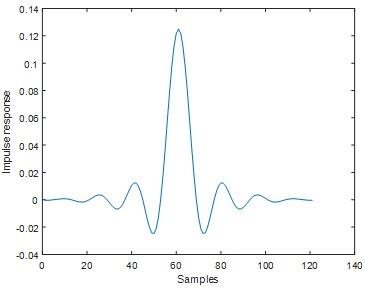Fig 8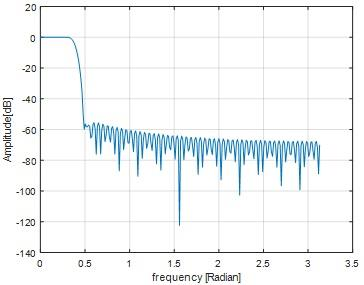Fig 9

Hanning window result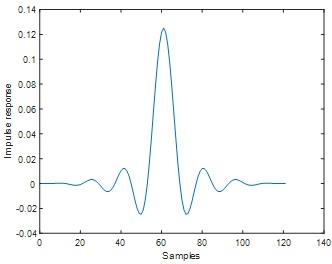Fig 10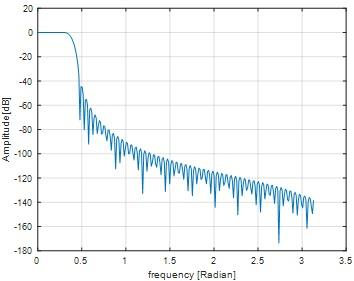Fig 11

In both hamming and hanning window ripple is not exist.Stop band attenuation of hamming window is approximately equal to -60 dB and for hanning window Stop band attenuation is approximately equal to -44 dB. From these results we inferred, For the given filter length hamming window yields lesser stop band attenuation comparing to hanning window.

NEVER MISS YOUR CHANCE TO EXCEL IN ME606 Z-TRANSFORMS AND FILTERS CONCEPTS ASSIGNMENT! AVAIL AFFORDABLE AND RELIABLE ME606 DIGITAL SIGNAL PROCESSING ASSIGNMENTS HELP SERVICES OF EXPERTSMINDS.COM

Question 6) Change ωp=pi/4 and repeat 1) and discuss the effect.

MATLAB code

wp=pi/4; % lowpass filter bandwidth

M=121;

n=-(M-1)/2:(M-1)/2; % selection time window

h0=(wp/pi)*sinc((wp/pi)*n); % truncated impulse response

h=h0.*rectwin(M)'; % windowing

% h=h0.*hamming(M)';% windowing

% h=h0.*hanning(M)';% windowing

% h=h0.*bartlett(M)';% windowing

% h=h0.*blackman(M)';% windowing

figure;plot(h)

ylabel('Impulse response')

xlabel('Samples')

% spectrum

FFTsize=512;

pxx=20*log10(abs(fft(h,FFTsize)));

fxx=(0:(FFTsize/2)-1)*(pi/(FFTsize/2));

figure;plot(fxx,pxx(1:FFTsize/2));

ylabel('Amplitude[dB]');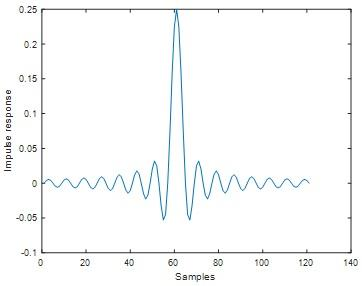Fig 12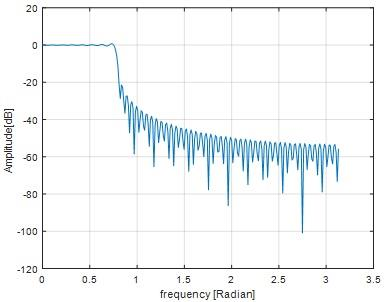Fig 13

If we change the value of omega from pi/8 to pi/4 , Amplitude of the impulse response is increases,pass band and stop band frequency also increases.

1 -2 Designing a bandpass FIR filter using Windowed Fourier Series approach

Question 7) Run the above program and plot the impulse response and the amplitude spectrum of the filter.

MATLAB code

wp=pi/16; % lowpass filter bandwidth

M=121;

n=-(M-1)/2:(M-1)/2; % selection time window

h0=(wp/pi)*sinc((wp/pi)*n); % truncated impulse response

h1=h0.*rectwin(M)'; % windowing

w0=pi/4; % BPF center frequency

h=h1.*exp(j*w0*n); % Lowpass to bandpass conversion

figure; subplot(211);plot(real(h));ylabel('Real part of impulse response')

xlabel('samples')

subplot(212);plot(imag(h));ylabel('imag part of impulse response')

xlabel('samples') % spectrum

FFTsize=512;

pxx=20*log10(abs(fft(h,FFTsize)));

fxx=(0:(FFTsize/2)-1)*(pi/(FFTsize/2));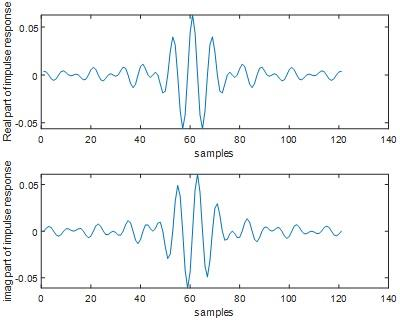Fig 14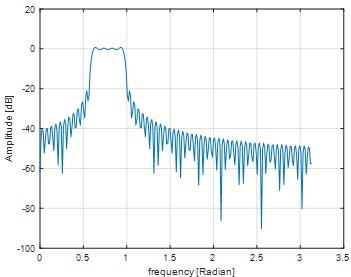Fig 15

Question 8) What are the passband edges ωp1 ωp2. Measure the attenuation in frequency response at these frequencies and compare them with the expected value of -6dB.

Passband Edges

ωp1=0.53

ωp2=1

Attenuation using ωp1

A1=-20log10(ωp1)

A1=-20log10(0.53)

A1=5.5145

Attenuation using ωp2

A2=-20log10(1)

A2=-20log10(1)

A2=0

The attenuation of the passband edge ωp1 is nearly equal to 6 dB. The attenuation of the  passband edge ωp2 is 0 dB which is not equal to 6 dB.

Question 9) Measure the maximum ripple in the passband.

Maximum ripple in the passband=0.01 dB

WE HELP STUDENTS TO IMPROVE THEIR GRADES! AVAIL TOP QUALITY ME606 Z-TRANSFORMS AND FILTERS CONCEPTS ASSIGNMENT HELP AND HOMEWORK WRITING SERVICES AT CHEAPER RATE!

Question 10) Increase ωp to pi/4 and discuss the result

MATLAB code

wp=pi/4; % lowpass filter bandwidth

M=121;

n=-(M-1)/2:(M-1)/2; % selection time window

h0=(wp/pi)*sinc((wp/pi)*n); % truncated impulse response

h1=h0.*rectwin(M)'; % windowing

w0=pi/4; % BPF center frequency

h=h1.*exp(j*w0*n); % Lowpass to bandpass conversion

figure; subplot(211);plot(real(h));ylabel('Real part of impulse response')

xlabel('samples')

subplot(212);plot(imag(h));ylabel('imag part of impulse response')

xlabel('samples') % spectrum

FFTsize=512;

pxx=20*log10(abs(fft(h,FFTsize)));

fxx=(0:(FFTsize/2)-1)*(pi/(FFTsize/2));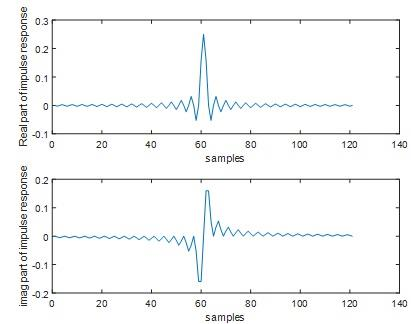Fig 16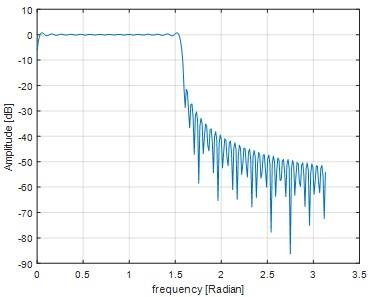Fig 17

When the frequency of ωp is increased into pi/4, the passband edges lying between 0.1 to 1.6.The transition bandwith also increased.The ripple remains same like before.

ENDLESS SUPPORT IN ME606 Z-TRANSFORMS AND FILTERS CONCEPTS ASSIGNMENTS WRITING SERVICES - YOU GET REVISED OR MODIFIED WORK TILL YOU ARE SATISFIED WITH OUR ME606 DIGITAL SIGNAL PROCESSING ASSIGNMENT HELP SERVICES!

1 -3 Designing a band-stop FIR filter using Frequency sampling approach

Question 11) Report your MATLAB code. Select the filter impulse response length M=121;

MATLAB code

wp=pi/8; % filter bandwidth

M=121;

n=-(M-1)/2:(M-1)/2; % selection time window

h0=(wp/pi)*sinc((wp/pi)*n); % truncated impulse response

h1=h0.*rectwin(M)'; % windowing

w0=pi/4; % BPF center frequency

h=h1-(h1.*exp(j*w0*n)); %band stop filter

figure; subplot(211);plot(real(h));ylabel('Real part of impulse response')

xlabel('samples')

subplot(212);plot(imag(h));ylabel('imag part of impulse response')

xlabel('samples')

FFTsize=512;

pxx=20*log10(abs(fft(h,FFTsize)));

fxx=(0:(FFTsize/2)-1)*(pi/(FFTsize/2));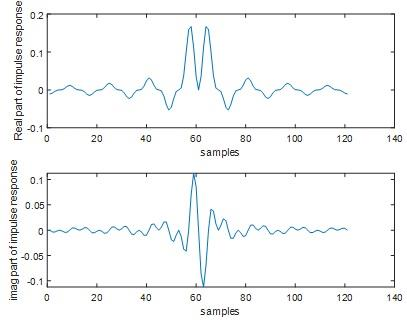Fig 18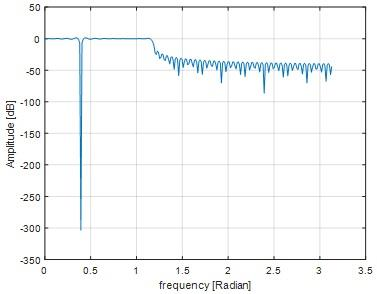Fig 19

Question 12) Plot the frequency response, |H(ejω)|, of your filter on the top of the spectrum mask in a single plot.

MATLAB code

bs = fdesign.bandstop('Fp1,Fst1,Fst2,Fp2,Ap1,Ast,Ap2', ...

pi/4,pi/3.8,pi/3.5,1,1,60,1);

bs1 = design(bs);

freqz(bs1);

[fp wp]=freqz(bs1);

figure

plot(wp/pi,db(fp));

ylabel('|H(e^(j?))|')

title('Frequency Response')

grid on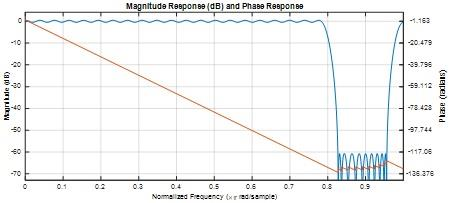Fig 20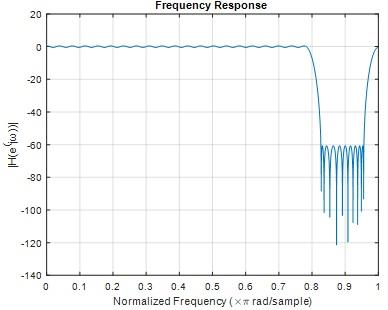Fig 21

Question 13) Plot 20*log10(|H(ejω)|) of your filter on the top of the spectrum mask in dB in a single plot.

MATLAB code

bs = fdesign.bandstop('Fp1,Fst1,Fst2,Fp2,Ap1,Ast,Ap2', ...

pi/4,pi/3.8,pi/3.5,1,1,60,1);

bs1 = design(bs);

freqz(bs1);

[fp wp]=freqz(bs1);

figure

plot(wp/pi,20*log10(db(fp)));

ylabel('|H(e^jw)| in dB')

title('Frequency Response')

grid on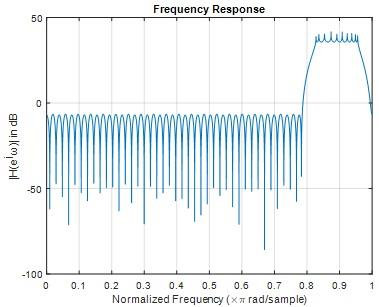Fig 22

Question 14) Measure the amplitude at ω = [Π/4, 3Π/4, Π/2] on your second plot.

MATLAB code

wp=pi/8; % lowpass filter bandwidth

M=121;

n=-(M-1)/2:(M-1)/2; % selection time window

h0=(wp/pi)*sinc((wp/pi)*n); % truncated impulse response

h1=h0.*rectwin(M)'; % windowing

w0=(pi)/4; % BPF center frequency

h=h1-(h1.*exp(j*w0*n)); %band stop filter

FFTsize=512;

pxx=20*log10(abs(fft(h,FFTsize)));

fxx=(0:(FFTsize/2)-1)*(pi/(FFTsize/2));

wp=pi/8; % lowpass filter bandwidth

M=121;

n=-(M-1)/2:(M-1)/2; % selection time window

h0=(wp/pi)*sinc((wp/pi)*n); % truncated impulse response

h1=h0.*rectwin(M)'; % windowing

w0=(3*pi)/4; % BPF center frequency

h=h1-(h1.*exp(j*w0*n)); %band stop filter

FFTsize=512;

pxx=20*log10(abs(fft(h,FFTsize)));

fxx=(0:(FFTsize/2)-1)*(pi/(FFTsize/2));

wp=pi/8; % lowpass filter bandwidth

M=121;

n=-(M-1)/2:(M-1)/2; % selection time window

h0=(wp/pi)*sinc((wp/pi)*n); % truncated impulse response

h1=h0.*rectwin(M)'; % windowing

w0=(pi)/2; % BPF center frequency

h=h1-(h1.*exp(j*w0*n)); %band stop filter

FFTsize=512;

pxx=20*log10(abs(fft(h,FFTsize)));

fxx=(0:(FFTsize/2)-1)*(pi/(FFTsize/2));

Omega=pi/4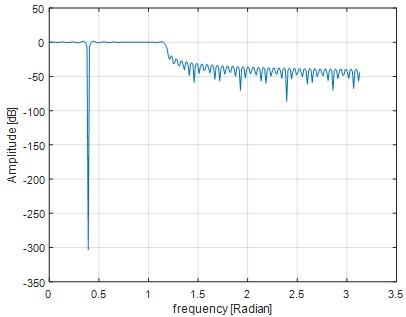Fig 23

Omega=3*pi/4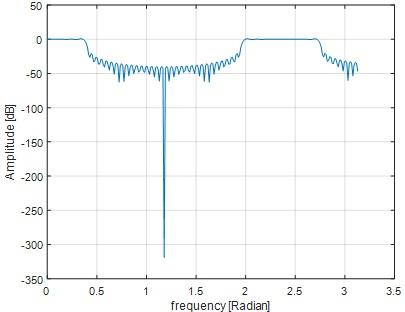Fig 24

Omega=pi/2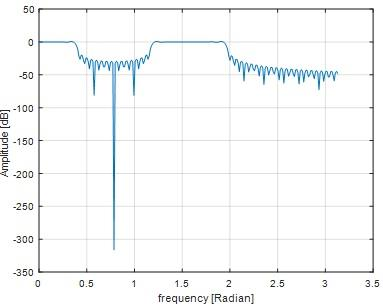Fig 25

Question 15) Find the deepest point in your frequency response in dB. How you can increase the depth of the stopband?

Deepest point in frequency response is 0.88.By increasing the value of cutoff frequency we can increase the depth of stop band.

1-4 Designing a low pass FIR filter using Frequency sampling approach

Question 16) Run the above program and plot the impulse response and the amplitude spectrum of the filter. Try to understand what the program does.

MATLAB code

wp=pi/8;

M=121;

FFTsize=512; % passband frequency samples

Np=fix((wp/(2*pi))*FFTsize); % number of pass band samples

Ns=FFTsize/2-Np; % number of stop band samples

H1=[ones(1,Np) zeros(1,Ns+1)];

H=[H1 H1(end:-1:2)]; % sampled frequency spectrum;

fxx=(0:(FFTsize/2)-1)*(pi/(FFTsize/2));

figure;plot(fxx,H(1:FFTsize/2));

% finding impulse response by truncating

h1=real(ifft(H));

h0=[ h1(end-(M-1)/2+1:end) h1(1:1+(M-1)/2)];

figure;plot(h0)

ylabel('Impulse response')

xlabel('Samples')

% spectrum

pxx=20*log10(abs(fft(h0,FFTsize)));

fh=figure;plot(fxx,pxx(1:FFTsize/2));hold on;

% effect of windowing

h=h0.*hanning(M)';% windowing

% spectrum

pxx=20*log10(abs(fft(h,FFTsize)));

figure(fh);plot(fxx,pxx(1:FFTsize/2));grid on;   legend('rectwin','hanning')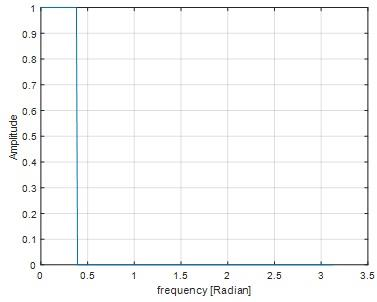Fig 26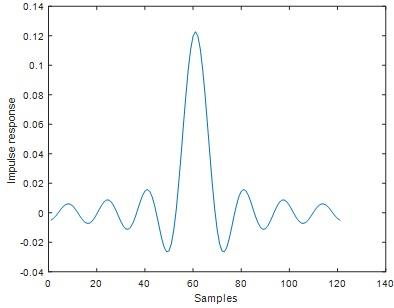Fig 27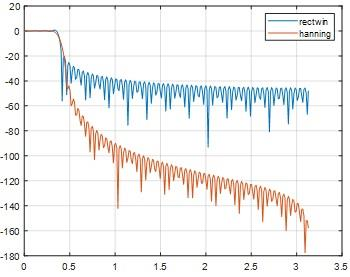Fig 28

Question 17) Use the above program as your base, design a band pass filter that passes the frequencies between pi/8<w<pi/4. Only modify the lines 5-7 and do not touch the rest of the program.

MATLAB code

wp=pi/8;

wp1=pi/4;

M=121;

FFTsize=512; % passband frequency samples

Np=fix((wp/(2*pi))*FFTsize); % number of pass band samples

Ns=fix((wp1/(2*pi))*FFTsize); % number of stop band samples

H1=[zeros(1,Np) ones(1,Ns+1) zeros(1,Np) ];

H=[H1 H1(end:-1:2)]; % sampled frequency spectrum;

fxx=(0:(FFTsize/2)-1)*(pi/(FFTsize/2));

figure;plot(fxx,H(1:FFTsize/2));

% finding impulse response by truncating

h1=real(ifft(H));

h0=[ h1(end-(M-1)/2+1:end) h1(1:1+(M-1)/2)];

figure;plot(h0)

ylabel('Impulse response')

xlabel('Samples')

% spectrum

pxx=20*log10(abs(fft(h0,FFTsize)));

fh=figure;plot(fxx,pxx(1:FFTsize/2));hold on;

% effect of windowing

h=h0.*hanning(M)';% windowing

% spectrum

pxx=20*log10(abs(fft(h,FFTsize)));

figure(fh);plot(fxx,pxx(1:FFTsize/2));grid on;   legend('rectwin','hanning')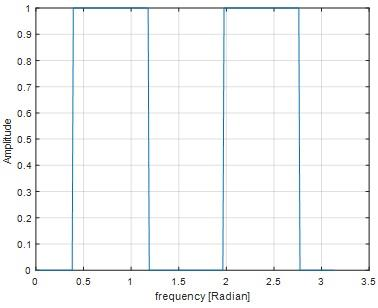Fig 29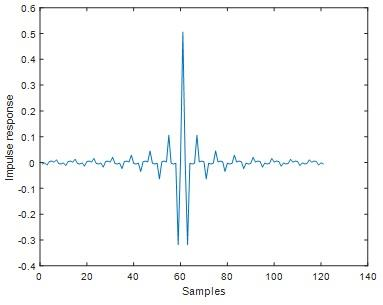Fig 30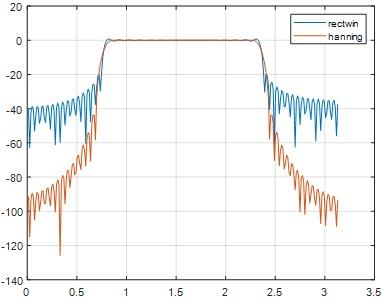Fig 31

EXPERTSMINDS.COM GIVES ACCOUNTABILITY OF YOUR TIME AND MONEY - AVAIL TOP RESULTS ORIGINATED ME606 DIGITAL SIGNAL PROCESSING ASSIGNMENT HELP SERVICES AT BEST RATES!

Section 2. Analyzing a system in Z and time domain

Question 18) Assume the system is causal. Draw its region of convergence (ROC) in the z-plane

MATLAB code

nm=[1 -0.2];

dm=[1 -0.8 0.15]

[a b c]=tf2zp(nm,dm);

zplane(nm,dm);

H(z)=(1-0.2z^(-1))/((1-0.5z^(-1) )(1-0.3z^(-1)))

H(z)=(1-0.2z^(-1))/((1-0.3z^(-1)-0.5z^(-1) )+0.15z^(-2)))

H(z)=(1-0.2z^(-1))/((1-0.8z^(-1) )+0.15z^(-2)))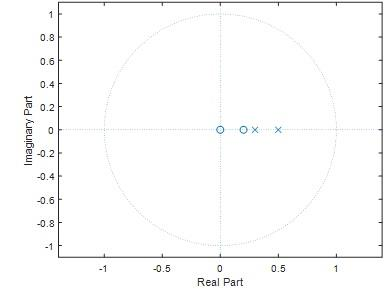Fig 32

Question  19) Is the system stable or unstable? Why?

The given system is stable.From the Fig 32 we can see that all the poles are inside the unit circle. Therefore the system is said to be stable.

Question 20) Determine the system difference equation in the form of

k=0N akY[n-k] = ∑k=0M bkx[n-k]

H(z)=(1-0.2z^(-1))/((1-0.8z^(-1) )+0.15z^(-2)))

H(z)=Y(z)/X(z)

Y(z)/X(z)=(1-0.2z^(-1))/((1-0.8z^(-1) )+0.15z^(-2)))

(1-0.8z^(-1)+0.15z^(-2) )Y(z)=(1-0.2z^(-1)) X(z)

Y(z)-0.8z^(-1) Y(z)+0.15z^(-2) Y(z)=X(z)-0.2z^(-1) X(z)

u(n)-0.8y(n-1)+0.15y(n-2)=u(n)-0.2x(n-1)

0.8y(n-1)-0.15y(n-2)=0.2x(n-1)

-5.3[y(n-1)+y(n-2)]=-1.3x(n-1)

∑_(k=0)^2?y(n-k)=? ∑_(k=0)^1x(n-k)

Question  21) Give the values for ai and bj , N and M

ai=-5.3
bi=-1.3

N=2

M=1

Question 22) Sketch the magnitude of the frequency response of the system between 0 and Π

MATLAB code

b=[1 -0.2];

a1=[1 -0.5];

a2=[1 -0.3];

ak=conv(a1,a2);

freqz(b,ak,1000)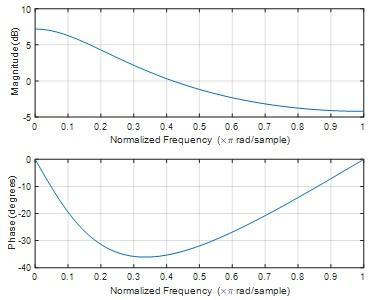Fig 33

Question 23) Suppose the above transfer function is a filter, what type of filter is it?

Low pass filter

Question 24) Sketch a block diagram for implementing this system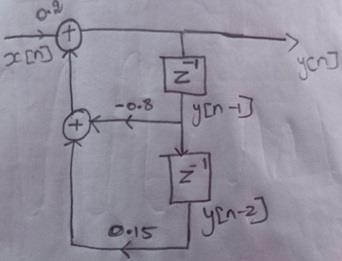Question Answer 25) Find the system causal impulse response

MATLAB code

nm=[1 -0.2];

dm=[1 -0.8 0.15]

[p q r]=residue(nm,dm)

gg=tf(p',q');

figure

impulse(gg)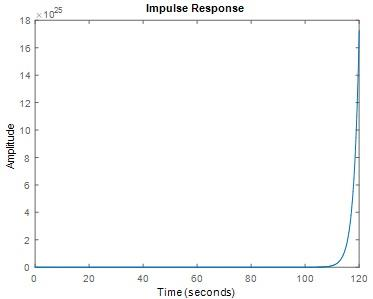Fig 34

Question 26) Assume the initial condition is zero, enter a unit impulse to the system and find the impulse response of the system h[n] for the first 10 samples and compare it with the step11

MATLAB code

nm=[1 -0.2];

dm=[1 -0.8 0.15]

figure

impz(nm,dm)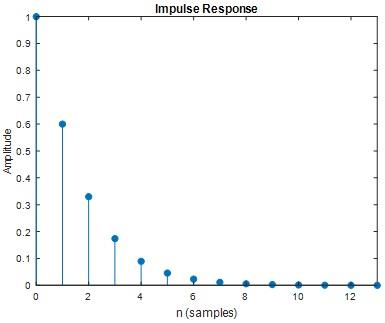Fig 35

Section 3. Filtering

Question 27) Plot and include the plot in your report. Simply, mention the application of the "pwelch" program, and what are its input parameters.

MATLAB code

x=randn(1,5000); % signal to be filtered

NFFT=512; % FFTT size (N)

overlap_samples=0; % number of overlap samples in time frames

y=conv(x,h); % filtering operation using convolution

[Pxx,W] = pwelch(y,hamming(NFFT),overlap_samples,NFFT);

figure;plot(W,Pxx);

xlabel('W [radian] from 0-pi');ylabel(' power spectral density')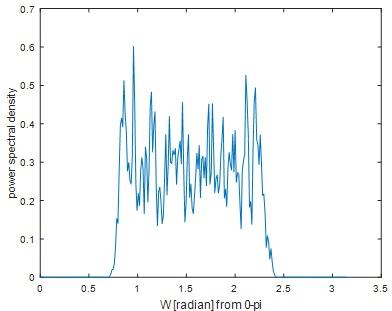Fig 36

Application of Pwelch

signal simulation and measurements

noise simulation and measurements

In the field of dividing  signal into segments

Input parameters of Pwelch

1.       Input signal

2.       Window

3.       Noverlap samples

4.       Number of DFT points

Question 28) Measure the filter delay and find a ratio of the filter delay to the filter impulse response length

MATLAB code

x=[-zeros(1,500) ones(1,1000) zeros(1,500)]; % signal to be filtered

y=conv(x,h); % filtering operation using convolution

figure;plot(x,'.-')

hold on;

plot(y,'.-'); grid; xlabel('sample')

legend('filter input signal','filter output')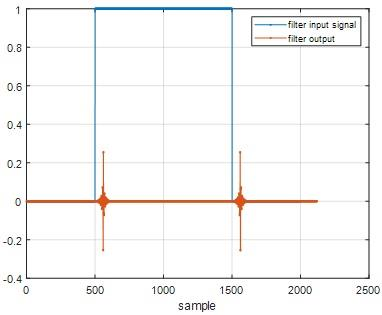Fig 37

From fig 37 we can obtain,

Filter delay= 0.23 secs

Filter impulse response length=1000

Ratio= Filter delay/ Filter impulse response length =2.3*10-4

SAVE DISTINCTION MARKS IN EACH ME606 Z-TRANSFORMS AND FILTERS CONCEPTS ASSIGNMENT WHICH IS WRITTEN BY OUR PROFESSIONAL WRITER!

Get our Melbourne Institute of Technology Assignment Help for the below mentioned courses like:-

• ME700 Industry Experience Assignment Help
• MN506 System Management Assignment Help
• ME603 Project Assignment Help
• MN503 Overview of Internetworking Assignment Help
• MN605 Enterprise Network Design Assignment Help
• ME503 Telecommunication System Engineering Assignment Help
• ME501 Professional Engineering Practice Assignment Help
• ME602 Mobile and Satellite Communication Systems Assignment Help
• ME693 Software-Defined Radio Communication Assignment Help
• ME601 Telecommunication Modeling and simulation Assignment Help
Tag This :- EM201925SAI530EE, ME606 Z-Transforms and Filters Concepts Assignment Help### Assignment Samples

 ITC544 IT Fundamentals Assignment Help itc544 it fundamentals assignment help - Investigate and describe the essential elements of a computer and their functionalities. MGMT495 Business Capstone Assignment Help mgmt495 business capstone assignment help-Colorado Technical University - Prepare a case study on an energy company to establish if it is a good choice. Cyclic Redundancy Check Assignment Help cyclic redundancy check assignment help - draw the 16 qam constellation diagram having two different amplitude levels and eight different phase levels Social Change Assignment Help social change assignment help - You will explore how your program might influence social change in a community BN106 Networking Fundamentals Assignment Help bn106 networking fundamentals assignment help - melbourne institute of technology - recognize network topologies, media types and network components. Issues in Environmental and Occupational Health Assignment Help issues in environmental and occupational health assignment help - this paper includes information regarding all aspects which should be covered in the priority MEM234026A Develop and Coordinate Engineering - Related Contingency Plans Assignment Help mem234026a develop and coordinate engineering - related contingency plans assignment help - establish contingency plan task. review existing technologies.Get Academic Excellence with Best Skilled Tutor! Order Assignment Now!